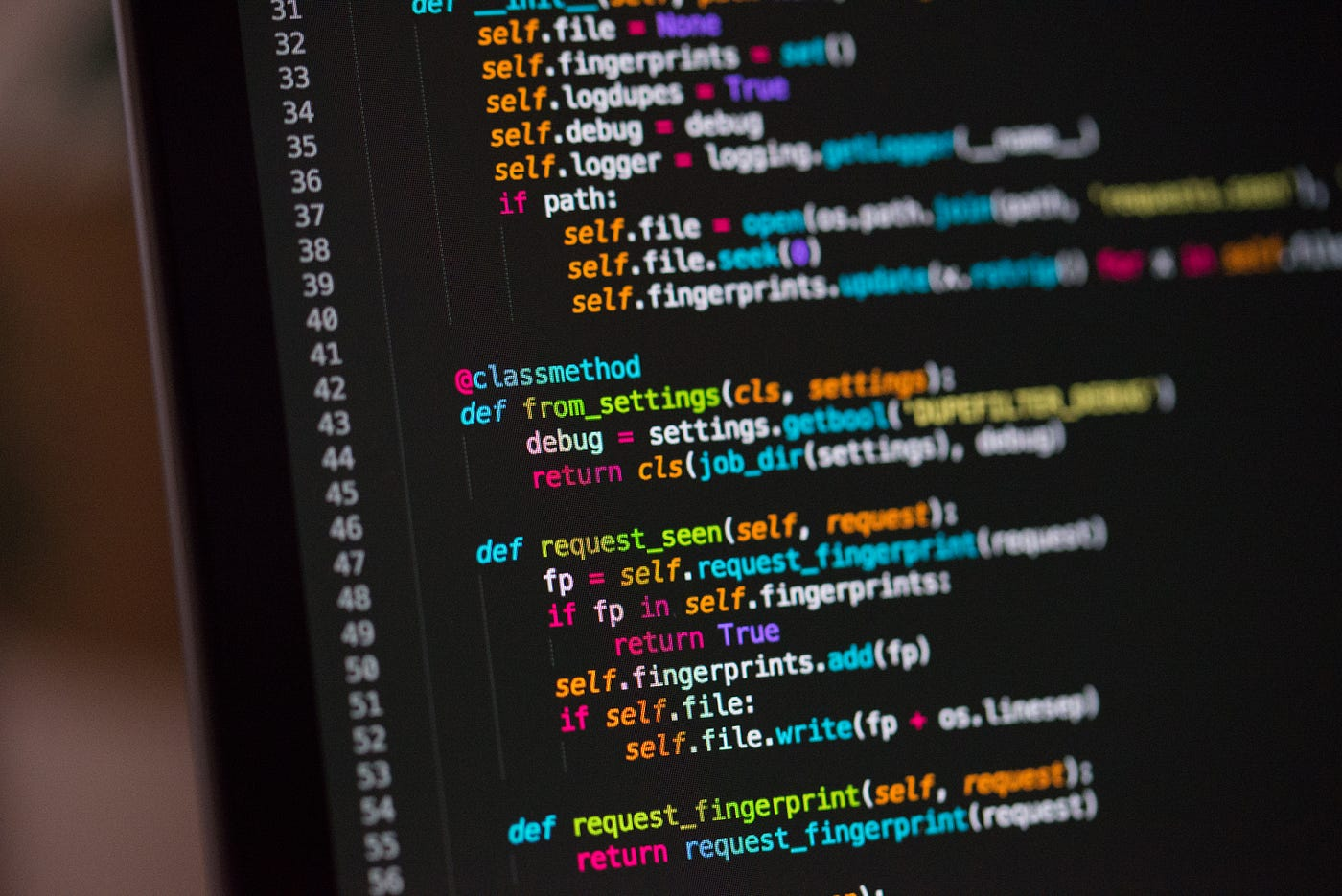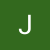# The Blind 75 Leetcode Series: Set Matrix ZeroesPhoto by Chris Ried on Unsplash

Today, we are working on 73. Set Matrix Zeroes

Given an `m x n` integer matrix `matrix`, if an element is `0`, set its entire row and column to `0`'s.

You must do it in place.

We are given a matrix with elements in it. If we ever see a `0`, we replace the entire row and entire column of that 0 with `0`’s. We have to modify the matrix directly instead of creating a new matrix.

Some things to clarify would be:

1. are we expecting empty matrix for a test case?
2. What are the element types we are expecting?
3. are the 0’s we want to identify only in `int`?

If the interviewers tell us that the matrix always hold some values, and the values are all integers, then we know that

Given an `m x n` non-empty integer matrix `matrix`, if an element is `0`, set its entire row and column to `0`'s.

You must do it in place.

We can now work on it.

Since we are requested to do it in place, we have to modify the matrix itself. In order to do that, we have to make sure we only change correct elements to 0s. This means when we change elements to 0s, we have to make sure we don’t count them again for change its row and column to zeros as well.

One straightforward solution would be using a tracker to track the rows and columns we need to change as we traverse through the matrix. At the end, we go through each of the rows and columns and replace the elements with 0s so we can make sure we don’t double count.

`def setZeroes(matrix):    # trackers for which rows and cols to flip to 0s    rows, cols = set(), set()    m, n = len(matrix), len(matrix)    # first go through the matrix and find all 0s    for i in range(m):        for j in range(n):            # track which row and column            if matrix[i][j] == 0:                rows.add(i)                cols.add(j)    # go through each element in the trackers, change elements to 0s    while rows:        next_row = rows.pop()        matrix[i][:] = *n    while cols:        next_col = cols.pop()        for i in range(m):            matrix[i][next_col] = 0    # not needed for leetcode, but we just return the original matrix    return matrix`

What’s the time complexity? We are going through the matrix once, so that’s O(m*n) where m and n are length and width of the matrix. Then we go through each of the trackers THEN visit each element of the row/column to modify the element. Potentially we go through the entire matrix more times, so the overall time complexity becomes O(c*m*n) where c is some constant. The Big O at the end is still O(m*n).

We actually cannot improve the time complexity since we always have to go through the matrix at least once to identify the 0s and go through the elements to modify them to 0s. These operations are all proportional to the length and width of the matrix.

But for the sake of improving the solution, let’s work on the space complexity. Space complexity, which I’ve been ignoring, deals with extra spaces needed for a solution. In our first approach, we store the rows and columns that we are going to modify, so our space complexity is proportional to (m+n), which makes the complexity O(m+n).

How do we make it better?

The complexity better than linear is either logarithmic (O(logn))or constant (O(1)). Logarithmic complexity often deals with binary search (or cutting the problem in half and only having to deal with one half), which doesn’t apply for our problem, so we go for constant complexity.

We still need to track which rows and columns we want to modify, but we cannot use extra memory like set(). What do we do? We can only use the existing things: the matrix itself.

Modifying the matrix itself as tracker has the same risk as modifying the matrix as we go: we might change elements to zeros when we are not supposed to.

Let’s use the first row and first column as the tracker. If matrix[i][j] == 0, we mark matrix[j] = 0 and matrix[i] = 0. Once we have gone through the entire matrix, we go back and visit the first row and first column to determine which row/column we want to change to 0s. However, because we are modifying the first row and column, we want to make sure we have a way to track the original 0s in the first row/column.

For first column, we know the matrix will be set to 0, and even if matrix is originally 0, the first column should still be 0s, so we can simply check that.

For first row, it’s more complicated. We don’t have an element we can refer to as the indicator, so we want to use an extra variable, say, `first_row`, as indicator. If we see a zero in the original matrix in first row, we mark `first_row` as 1, otherwise `first_row` = 0.

Now we should have everything we need. To put it in code:

`def setZeroes(matrix):        m, n = len(matrix), len(matrix)    # first-row-has-0 indicator    first_row = 0    for i in range(m):        for j in range(n):            if matrix[i][j] == 0:                matrix[j] = 0                if i == 0:                    # first row                    first_row = 1                else:                    matrix[i] = 0    # for everything other than first row    for i in range(1, m):        if matrix[i] == 0:            matrix[i] =  * n    # for everything other than first column    for j in range(1, n):        if matrix[j] == 0:            for i in range(m):                matrix[i][j] = 0    # for first column    if matrix == 0:        for i in range(m):            matrix[i] = 0    # for first row    if first_row:        matrix =  * n`

For this approach, the only extra variable is `first_row`, and we track the rest in `matrix` itself.

I personally don’t care that much when dealing with whiteboarding problems like this. If you come up with the first approach, that’s good enough. It’s always a plus to have used the O(1) space, but it’s not a pass/fail situation for me as long as you can explain clearly when you decide to take such approach.

That’s it! Another problem down.